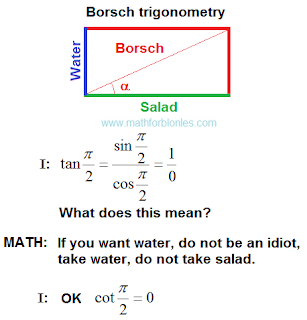## 11/02/2019

I will not tell you recipes for cooking borsch, I will talk about mathematics. What is borsch? These are vegetables cooked in water according to a specific recipe. I will consider two source components (vegetable salad and water) and the final result is borsch. Geometrically, this can be thought of as a rectangle in which one side is a salad, the other side is water. The sum of these two sides is borsch. The diagonal and area of such a rectangle are mathematical concepts and are never used in recipes.Borsch trigonometry

How do salad and water turn into borsch? How does the sum turn into trigonometry? To understand this, linear angular functions will help us.

The linear angular functions

There are no linear angular functions in mathematics textbooks. But without them there can be no mathematics. Mathematical laws work whether we know them or not.

The linear angular functions are the laws of addition. This is how algebra turns into geometry, and geometry turns into trigonometry.Trigonometry addition

The main law of addition, which mathematicians do not like to talk about, requires that the summands have the same units of measurements. For salad, water and borsch it can be units of weight, volume, value or others.Law of addition

The figure shows two levels of difference. The first level is differences in the field of numbers. This is what mathematicians do. The second level is the difference in the area of units of measurement (shown in square brackets). Physicists are doing this. We can understand the third level - the differences in the field of described objects. Different objects can describe the same number of identical units of measurements. How important this is, the trigonometry of borscht will show us. Here is how linear angular functions for borsch will look.The law of addition for borsch

Part of the water and part of the salad give one serving of borsch. Now we can see what will happen when the angle of linear angular functions changes.The angle is zero

The angle is zero. We have a salad but no water. You can’t cook borsch. The amount of borsch is also zero. This does not mean that zero borsch is equal to zero water. Zero borsch can be at zero salad (right angle).The angle is greater than zero, but less than the right angle

The angle is greater than zero, but less than forty-five degrees. We have a lot of salad, but not enough water. The result is a thick borsch.

The angle is forty-five degrees. We have an equal amount of water and lettuce. Perfect borsch (forgive me the cook, it's just math).

The angle is more than forty-five degrees, but less than ninety degrees. We have a lot of water and a little salad. Get liquid borsch.Right angle

Right angle. We have water. From the salad there were only memories. We cannot cook borscht. The amount of borscht is zero. In this case, drink water.

Something like this. If you're tired of borscht, check out a few other stories.

Percentage.Percentage

Cell division.Cell division

Two friends had their shares in the general business. After killing one of them, everything went to another.The general business

The emergence of mathematics on our planet.The emergence of mathematics

All these stories in the language of mathematics are presented using linear angular functions. I will tell you separately about the real place of these functions in the structure of mathematics. In the meantime, we return to the trigonometry of borscht and consider the projections.